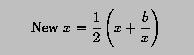# Computing Square Roots with Newton's Method### Problem Statement

We have discussed Newton's Method for computing the square root of a positive number. Let the given number be b and let x be a rough guess of the square root of b. Newton's method suggests that a better guess, New x can be computed as follows:One can start with b as a rough guess and compute New x; from New x, one can generate a even better guess, until two successive guesses are very close. Either one could be considered as the square root of b.

Write a function MySqrt() that accepts a formal argument and uses Newton's method to computes its square root. Then, write a main program that reads in an initial value, a final value, and a step size, and computes the square roots of these successive values with Newton'e method and Fortran's SQRT() function, and determines the absolute error.### Solution

```! ---------------------------------------------------------------
! This program contains a function MySqrt() that uses Newton's
! method to find the square root of a positive number.  This is
! an iterative method and the program keeps generating better
! approximation of the square root until two successive
! approximations have a distance less than the specified tolerance.
! ---------------------------------------------------------------

PROGRAM  SquareRoot
IMPLICIT  NONE

REAL    :: Begin, End, Step
REAL    :: x, SQRTx, MySQRTx, Error

x = Begin                            ! x starts with the init value
DO
IF (x > End)  EXIT                ! exit if x > the final value
SQRTx   = SQRT(x)                 ! find square root with SQRT()
MySQRTx = MySqrt(x)               ! do the same with my sqrt()
Error   = ABS(SQRTx - MySQRTx)    ! compute the absolute error
WRITE(*,*)  x, SQRTx, MySQRTx, Error   ! display the results
x = x + Step                      ! move on to the next value
END DO

CONTAINS

! ---------------------------------------------------------------
! REAL FUNCTION  MySqrt()
!    This function uses Newton's method to compute an approximate
! of a positive number.  If the input value is zero, then zero is
! returned immediately.  For convenience, the absolute value of
! the input is used rather than kill the program when the input
! is negative.
! ---------------------------------------------------------------

REAL FUNCTION  MySqrt(Input)
IMPLICIT  NONE
REAL, INTENT(IN) :: Input
REAL             :: X, NewX
REAL, PARAMETER  :: Tolerance = 0.00001

IF (Input == 0.0) THEN            ! if the input is zero
MySqrt = 0.0                   !    returns zero
ELSE                              ! otherwise,
X = ABS(Input)                 !    use absolute value
DO                             !    for each iteration
NewX  = 0.5*(X + Input/X)   !       compute a new approximation
IF (ABS(X - NewX) < Tolerance)  EXIT  ! if very close, exit
X = NewX                    !       otherwise, keep the new one
END DO
MySqrt = NewX
END IF
END FUNCTION  MySqrt

END PROGRAM  SquareRoot
```
Click here to download this program.### Program Input and Output

If the input values of Begin, End and Step are 0.0, 10.0 and 0.5, the following is the output from the above program.
```0.E+0,  0.E+0,  0.E+0,  0.E+0
0.5,  0.707106769,  0.707106769,  0.E+0
1.,  1.,  1.,  0.E+0
1.5,  1.22474492,  1.2247448,  1.192092896E-7
2.,  1.41421354,  1.41421354,  0.E+0
2.5,  1.58113885,  1.58113885,  0.E+0
3.,  1.73205078,  1.7320509,  1.192092896E-7
3.5,  1.87082875,  1.87082863,  1.192092896E-7
4.,  2.,  2.,  0.E+0
4.5,  2.12132025,  2.12132025,  0.E+0
5.,  2.23606801,  2.23606801,  0.E+0
5.5,  2.34520793,  2.34520793,  0.E+0
6.,  2.44948983,  2.44948959,  2.384185791E-7
6.5,  2.54950976,  2.54950976,  0.E+0
7.,  2.64575124,  2.64575148,  2.384185791E-7
7.5,  2.73861289,  2.73861265,  2.384185791E-7
8.,  2.82842708,  2.82842708,  0.E+0
8.5,  2.91547585,  2.91547585,  0.E+0
9.,  3.,  3.,  0.E+0
9.5,  3.08220696,  3.08220696,  0.E+0
10.,  3.1622777,  3.1622777,  0.E+0
```### Discussion

This program has nothing special. Please refer to the discussion of Newton's method for the computation details of function MySqrt(). However, there is one thing worth to be mentioned. Since the formal argument Input is declared with INTENT(IN), it cannot be changed in function MySqrt(). Therefore, the value of the formal argument Input is copied to X and used in square root computation.